SSC CGL Previous Year Questions: Trigonometry - 5

# SSC CGL Previous Year Questions: Trigonometry - 5 - SSC CGL

Test Description

## 41 Questions MCQ Test SSC CGL (Tier - 1) - Previous Year Papers (Topic Wise) - SSC CGL Previous Year Questions: Trigonometry - 5

SSC CGL Previous Year Questions: Trigonometry - 5 for SSC CGL 2023 is part of SSC CGL (Tier - 1) - Previous Year Papers (Topic Wise) preparation. The SSC CGL Previous Year Questions: Trigonometry - 5 questions and answers have been prepared according to the SSC CGL exam syllabus.The SSC CGL Previous Year Questions: Trigonometry - 5 MCQs are made for SSC CGL 2023 Exam. Find important definitions, questions, notes, meanings, examples, exercises, MCQs and online tests for SSC CGL Previous Year Questions: Trigonometry - 5 below.
Solutions of SSC CGL Previous Year Questions: Trigonometry - 5 questions in English are available as part of our SSC CGL (Tier - 1) - Previous Year Papers (Topic Wise) for SSC CGL & SSC CGL Previous Year Questions: Trigonometry - 5 solutions in Hindi for SSC CGL (Tier - 1) - Previous Year Papers (Topic Wise) course. Download more important topics, notes, lectures and mock test series for SSC CGL Exam by signing up for free. Attempt SSC CGL Previous Year Questions: Trigonometry - 5 | 41 questions in 41 minutes | Mock test for SSC CGL preparation | Free important questions MCQ to study SSC CGL (Tier - 1) - Previous Year Papers (Topic Wise) for SSC CGL Exam | Download free PDF with solutions
 1 Crore+ students have signed up on EduRev. Have you?
SSC CGL Previous Year Questions: Trigonometry - 5 - Question 1

### The angles of elevation of the top of a tower standing on a horizontal plane from two points on a line passing through the foot of the tower at a distance 9 ft and 16 ft respectively are complementary angles. Then the height of the tower is    (SSC CGL 2nd Sit. 2012)

Detailed Solution for SSC CGL Previous Year Questions: Trigonometry - 5 - Question 1

In ΔABC
tan α = h/9 ... (1)
In ΔABD
tan β = h/16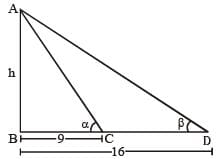α + β = 90° (given)
β = 90 – α
Since, tan β = h/16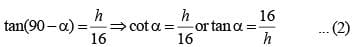From eqn. (1) and (2)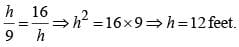SSC CGL Previous Year Questions: Trigonometry - 5 - Question 2

###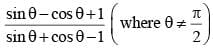is equal to   (SSC CGL 2nd Sit. 2012)

Detailed Solution for SSC CGL Previous Year Questions: Trigonometry - 5 - Question 2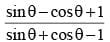Dividing Numerator and Denominator by cos θ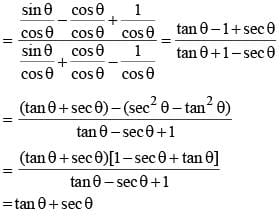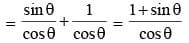SSC CGL Previous Year Questions: Trigonometry - 5 - Question 3

###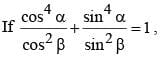then the value of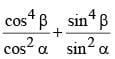is   (SSC CGL 2nd Sit. 2012)

Detailed Solution for SSC CGL Previous Year Questions: Trigonometry - 5 - Question 3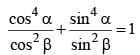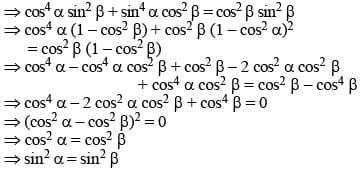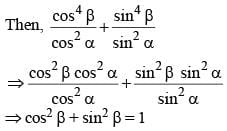SSC CGL Previous Year Questions: Trigonometry - 5 - Question 4

In a triangle ABC, AB = AC, BA is produced to D in such a manner that AC = AD. The circular measure of ∠BCD is    (SSC CGL 2nd Sit. 2012)

Detailed Solution for SSC CGL Previous Year Questions: Trigonometry - 5 - Question 4

AB = AC
∴ ∠ABC = ∠ACB ...(1)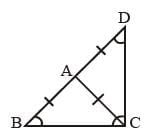[opposite angles of equal sides are equal]
AC = AD and BA = AC
∴ ∠ACD = ∠ADC and ∠ABC = ∠ACB ...(2)
In a triangle,
∠ABC + ∠ADC + ∠DCB = 180° (Angle sum property)
∠ABC + ∠ADC + ∠ACB + ∠ACD = 180°
2∠ACB + 2∠ACD = 180°
[From eqn. (1) & (2)]
∴ ∠BCD = 90° or π / 2

SSC CGL Previous Year Questions: Trigonometry - 5 - Question 5

The radian measure of 63°14'51" is   (SSC CGL 2nd Sit. 2012)

Detailed Solution for SSC CGL Previous Year Questions: Trigonometry - 5 - Question 5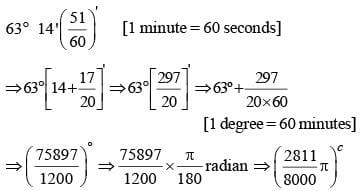SSC CGL Previous Year Questions: Trigonometry - 5 - Question 6

If sec θ + tan θ = √3, then the positive value of sin θ is    (SSC CGL 2nd Sit. 2012)

Detailed Solution for SSC CGL Previous Year Questions: Trigonometry - 5 - Question 6

sec2 θ – tan2 θ = 1
(sec θ + tan θ) (sec θ – tan θ) = 1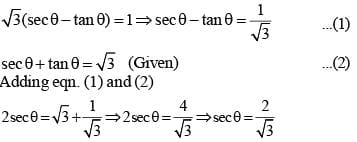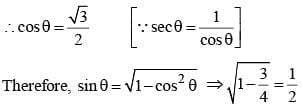SSC CGL Previous Year Questions: Trigonometry - 5 - Question 7

If 2y cos θ = x sin θ and 2x sec θ – y cosec θ = 3, then the relation between x and y is    (SSC CGL 2nd Sit. 2012)

Detailed Solution for SSC CGL Previous Year Questions: Trigonometry - 5 - Question 7

2y cos θ = x sin θ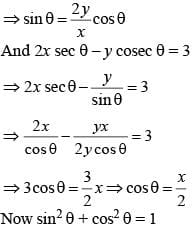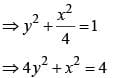SSC CGL Previous Year Questions: Trigonometry - 5 - Question 8

If sinθ + sin2θ = 1, then the value of cos12θ + 3cos10θ + 3cos8θ + cos6θ – 1 is    (SSC CGL 1st  Sit. 2012)

Detailed Solution for SSC CGL Previous Year Questions: Trigonometry - 5 - Question 8

sinθ + sin2θ = 1
⇒ sinθ = 1 – Sin2θ
⇒ sinθ = cos2θ
∴ cos12θ + 3cos10θ + 3cos8θ + cos6θ – 1
= (cos4θ + cos2θ)3 – 1
= (sin2θ + cos2θ)3 – 1 = 1 – 1 = 0

SSC CGL Previous Year Questions: Trigonometry - 5 - Question 9

When the angle of elevation of the sum increases from 30° to 60°, the shadow of a post is diminished by 5 metres. then the height of the post is    (SSC CGL 1st  Sit. 2012)

Detailed Solution for SSC CGL Previous Year Questions: Trigonometry - 5 - Question 9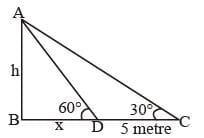AB = Pole = h metre
BD = x metre
From ΔABC,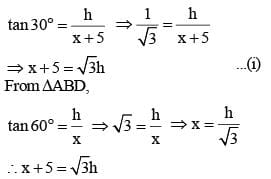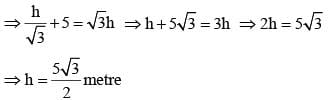SSC CGL Previous Year Questions: Trigonometry - 5 - Question 10

If 0 ≤ 2 ≤ π/2, 2y cosθ = x sin θ and 2x secθ – y cosecθ = 3, then the value of x2 + 4y2 is    (SSC CGL 1st  Sit. 2012)

Detailed Solution for SSC CGL Previous Year Questions: Trigonometry - 5 - Question 10

2y cos θ = xsin θ
⇒ x sec θ = 2y cosec θ
Also, 2x sec θ – y cosec θ = 3
⇒ 4y cosec θ – y cosec θ = 3
⇒ 3y cosec θ = 3
⇒ y cosec θ = 1
⇒ y = sin θ
∴ x sec θ = 2y cosec θ
= 2sin θ . cosec θ = 2
⇒ x = 2cos θ
∴ x2 + 4y2 = 4cos2θ + 4sin2θ = 4

SSC CGL Previous Year Questions: Trigonometry - 5 - Question 11

If x = cosecθ – sinθ and y = secθ – cosθ, then the value of x2y2 (x2 + y2 + 3) is     (SSC CGL 1st  Sit. 2012)

Detailed Solution for SSC CGL Previous Year Questions: Trigonometry - 5 - Question 11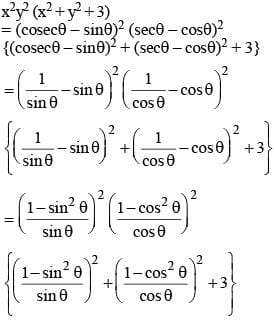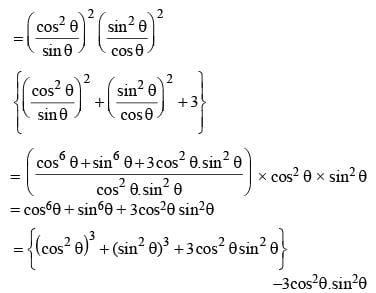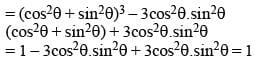SSC CGL Previous Year Questions: Trigonometry - 5 - Question 12

If tan (x + y) tan (x – y) = 1, then the value of tan (2x/3) is   (SSC CGL 1st  Sit. 2012)

Detailed Solution for SSC CGL Previous Year Questions: Trigonometry - 5 - Question 12

tan (x + y). tan (x – y) = 1
⇒ tan (x + y) = cot (x – y) = tan (90°– x + y)
⇒ x + y = 90° – x + y ⇒ 2x = 90°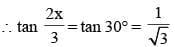SSC CGL Previous Year Questions: Trigonometry - 5 - Question 13

The least value of (4sec2θ + 9 cosec2θ) is    (SSC CGL 1st  Sit. 2012)

Detailed Solution for SSC CGL Previous Year Questions: Trigonometry - 5 - Question 13

4 sec2 θ + 9cosec2 θ
= 4 (1 + tan2 θ) + 9 (1 + cot2 θ)
= 4 + 4 tan2 θ + 9 + 9cot2 θ
= 4 tan2 θ + 9cot2θ +  12 – 12 + 13
= (2tan2 θ – 3Cot2 θ)2 + 25
{∵ least value of 2 tan2 θ – 3cot2 θ = 0}
∴ the minimum value is 25.

SSC CGL Previous Year Questions: Trigonometry - 5 - Question 14

If 5 tanθ = 4, then the value of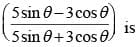(SSC CGL 1st  Sit. 2012)

Detailed Solution for SSC CGL Previous Year Questions: Trigonometry - 5 - Question 14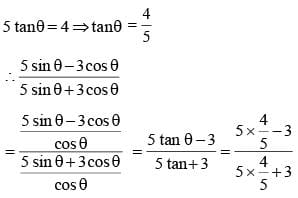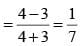SSC CGL Previous Year Questions: Trigonometry - 5 - Question 15

If x, y are positive acute angles, x + y < 90° and sin (2x – 20°) = cos (2y + 20°), then the value of sec (x + y) is    (SSC CGL 2nd  Sit. 2012)

Detailed Solution for SSC CGL Previous Year Questions: Trigonometry - 5 - Question 15

sin (2x – 20°) = cos (2y + 20°)
⇒ sin (2x – 20°) = sin (90° – 2y – 20°) = sin (70° – 2y)
⇒ 2x – 20° = 70° – 2y ⇒ 2 (x + y) = 90° ⇒ x + y = 45°
∴ sec (x + y) = sec 45° = √2

SSC CGL Previous Year Questions: Trigonometry - 5 - Question 16

If α is a positive acute angle and 2sinα + 15cos2α = 7, then the value of cotα is:   (SSC CGL 2nd  Sit. 2012)

Detailed Solution for SSC CGL Previous Year Questions: Trigonometry - 5 - Question 16

2sinα + 15cos2α = 7
⇒ 2 sinα + 15 (1 – sin2α) = 7
⇒ 2 sinα + 15 – 15 sin2α = 7
⇒ 15 sin2α – 2 sinα – 8 = 0
⇒ 15 sin2α – 12 sinα + 10 sinα – 8 = 0
⇒ 3 sinα (5 sinα – 4) + 2 (5 sinα – 4) = 0
⇒ (3 sinα + 2) ( 5 sinα – 4) = 0
⇒ 5 sinα – 4 = 0
⇒  sinα = 4/5
∴ cosecα = 5/4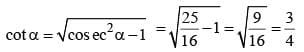SSC CGL Previous Year Questions: Trigonometry - 5 - Question 17

If (a2 – b2) sinθ + 2ab cosθ = a2 + b2, then the value of tanθ is    (SSC CGL 2nd  Sit. 2012)

Detailed Solution for SSC CGL Previous Year Questions: Trigonometry - 5 - Question 17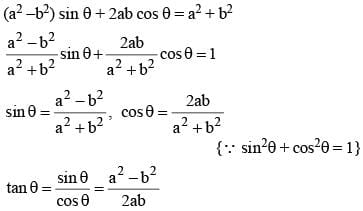SSC CGL Previous Year Questions: Trigonometry - 5 - Question 18

If tan θ – cot θ = a and cos θ – sin θ = b, then the value of (a2 + 4) (b2 – 1)2 is:   (SSC CGL 2nd  Sit. 2012)

Detailed Solution for SSC CGL Previous Year Questions: Trigonometry - 5 - Question 18

Put θ = 45°
a = tan 45° – cot 45°,  b = sin 45° – cos 45°
a = 1 – 1 = 0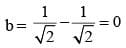Put in equation
(a2 + 4) (b2 –1)2 = (0 + 4) (0 – 1)2 = 4

SSC CGL Previous Year Questions: Trigonometry - 5 - Question 19

If a3 – b3 = 56 and a – b = 2, then the value of (a2 + b2) is:    (SSC CGL 2nd  Sit. 2012)

Detailed Solution for SSC CGL Previous Year Questions: Trigonometry - 5 - Question 19

(a – b)3 = a3 – b3 – 3ab (a – b)
⇒ 8 = 56 – 3ab (2)
⇒ 6ab = 56 – 8 = 48
⇒ 2ab = 16 ...(i)
∴ a2 + b2 = (a – b)2 + 2ab
= 4 + 16 = 20

SSC CGL Previous Year Questions: Trigonometry - 5 - Question 20

The simplified value of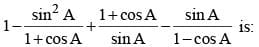(SSC CGL 2nd  Sit. 2012)

Detailed Solution for SSC CGL Previous Year Questions: Trigonometry - 5 - Question 20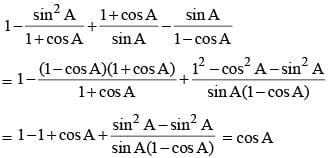SSC CGL Previous Year Questions: Trigonometry - 5 - Question 21

The least value of 4 cosec2α + 9sin2α is:   (SSC CGL 2nd  Sit. 2012)

Detailed Solution for SSC CGL Previous Year Questions: Trigonometry - 5 - Question 21

4 cosec2α + 9sin2α
= 4 cosec2α + 4 sin2α + 5 sin2α
= 4 [(cosecα – sinα)+ 2] + 5 sin2α
= 12  [∵ cosecα – sinα ≥ 1]

SSC CGL Previous Year Questions: Trigonometry - 5 - Question 22

If tan(x + y) tan (x – y) = 1, then the value of tan x is:    (SSC CGL 2nd Sit. 2012)

Detailed Solution for SSC CGL Previous Year Questions: Trigonometry - 5 - Question 22

tan (x + y) tan (x – y) = 1
⇒ tan (x + y) = cot (x – y) = tan (90° – (x – y))
⇒ x + y = 90°  – ( x – y)
⇒ 2x = 90°
⇒  x = 45°
∴ tan x = tan 45° = 1

SSC CGL Previous Year Questions: Trigonometry - 5 - Question 23

A boy standing in the middle of a field, observes a flying bird in the north at an angle of elevation of 30° and after 2 minutes, he observes the same bird in the south at an angle of elevation of 60°. If the bird flies all along in a straight line at a height of 50√3 m, then its speed in km/h is:    (SSC CGL 2nd Sit. 2012)

Detailed Solution for SSC CGL Previous Year Questions: Trigonometry - 5 - Question 23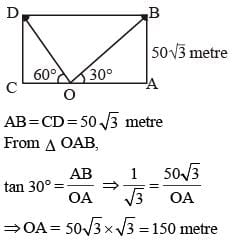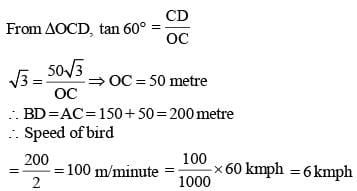SSC CGL Previous Year Questions: Trigonometry - 5 - Question 24

In a right-angled triangle ABC, ÐB is the right angle and AC = 2√5 cm. If AB – BC = 2 cm, then the value of (cos2A – cos2C) is:   (SSC CGL 2nd Sit.  2012)

Detailed Solution for SSC CGL Previous Year Questions: Trigonometry - 5 - Question 24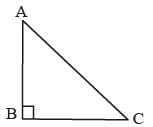Let BC = x
∴ AB = x + 2
∴ AB2 + BC2 = AC2
⇒ (x + 2)2 + x2 = (2√5) 2 ⇒ x2 + 4x + 4 + x2 = 20
⇒ 2x2 + 4x – 16 = 0
⇒ x2 + 2x – 8 = 0 ⇒ x2 + 4x – 2x – 8 = 0
⇒ x (x + 4)  – 2 (x + 4) = 0
⇒ (x – 2) (x + 4) = 0
⇒ x = 2 = BC
∴ AB = 2 + 2 = 4 cm

SSC CGL Previous Year Questions: Trigonometry - 5 - Question 25

If cot A + cosec A = 3 and A is an acute angle, then the value of cos A is:    (SSC CGL 2nd Sit. 2012)

Detailed Solution for SSC CGL Previous Year Questions: Trigonometry - 5 - Question 25

cot A +  cosec A = 3
cosec2 A – cot2 A = 1
(cosec A – cot A) (cosec A + cot A) = 1
cosec A – cot A = 1/3 ... (i)
cosec A + cot A = 3 ... (ii)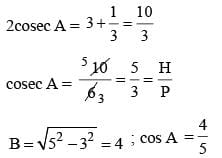SSC CGL Previous Year Questions: Trigonometry - 5 - Question 26

If cos A + cos2 A = 1, then sin2 A + sin4 A is equal to   (SSC CGL 1st Sit. 2012)

Detailed Solution for SSC CGL Previous Year Questions: Trigonometry - 5 - Question 26

cos A = 1 – cos2 A = sin2A
∴ sin2A + sin4A = sin2A + cos2A = 1

SSC CGL Previous Year Questions: Trigonometry - 5 - Question 27

A tree is broken by the wind. If the top of the tree struck the ground at an angle of 30° and at a distance of 30 m from the root, then the height of the tree is    (SSC CGL 1st Sit.2012)

Detailed Solution for SSC CGL Previous Year Questions: Trigonometry - 5 - Question 27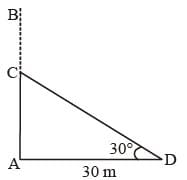AB = tree
BC = broken part
∴ BC = CD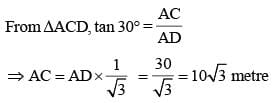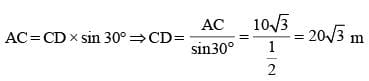∴ AB = AC + BC
= 10√3 + 20√3 = 30√3 metre

SSC CGL Previous Year Questions: Trigonometry - 5 - Question 28

sec4θ – sec2θ is equal to   (SSC CGL 1st Sit. 2012)

Detailed Solution for SSC CGL Previous Year Questions: Trigonometry - 5 - Question 28

sec4θ – sec2θ = sec2 θ (sec2θ – 1)
= (1 + tan2θ) (1 + tan2θ – 1) = tan2θ + tan4θ

SSC CGL Previous Year Questions: Trigonometry - 5 - Question 29

If x sin3 θ + y cos3 θ = sin θ cos θ and x sin θ = y cos θ, sin θ ≠ 0, cos θ ≠ 0, then x2 + y2 is        (SSC CGL 1st Sit. 2012)

Detailed Solution for SSC CGL Previous Year Questions: Trigonometry - 5 - Question 29

x sin3θ + y cos3θ = sinθ.cosθ
=> (x sinθ). sin2θ + (y cosθ) cos2θ = sinθ.cosθ
=> xsinθ . sin2θ + xsinθ . cos2θ = sinθ . cosθ
=> x sinθ (sin2θ + cos2θ) = sinθ . cosθ
=> x = cosθ
now, x sinθ = y cosθ
=> cosθ . sinθ = y cosθ
=> y = sinθ
x2 + y2 = cos2θ + sin2θ = 1

SSC CGL Previous Year Questions: Trigonometry - 5 - Question 30

If sin θ + cos θ = √2 cos (90° – θ), then cot θ is    (SSC CGL 1st Sit. 2012)

Detailed Solution for SSC CGL Previous Year Questions: Trigonometry - 5 - Question 30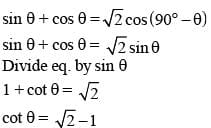SSC CGL Previous Year Questions: Trigonometry - 5 - Question 31

If 2 – cos 2θ = 3 sin θ cos θ, sin θ ≠ cos θ then tan θ is   (SSC CGL 1st Sit. 2012)

Detailed Solution for SSC CGL Previous Year Questions: Trigonometry - 5 - Question 31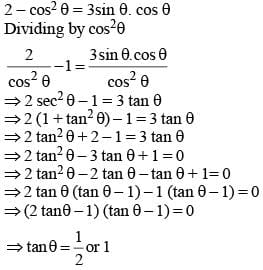SSC CGL Previous Year Questions: Trigonometry - 5 - Question 32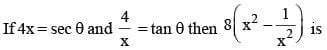(SSC CGL 1st Sit. 2012)

Detailed Solution for SSC CGL Previous Year Questions: Trigonometry - 5 - Question 32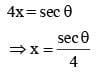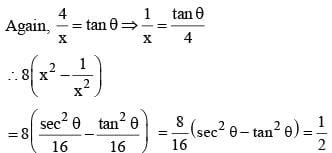SSC CGL Previous Year Questions: Trigonometry - 5 - Question 33

The least value of 2 sin2 θ + 3 cos2 θ is    (SSC CGL 1st Sit. 2012)

Detailed Solution for SSC CGL Previous Year Questions: Trigonometry - 5 - Question 33

2sin2 θ + 3 cos2 θ = 2 sin2 θ + 2cos2 θ + cos2 θ
= 2 (sin2θ + cos2θ) + cos2θ = 2 + cos2θ
∴ Least value = 2 + 0 = 2 [∵ cos2 θ ≥ 0]

SSC CGL Previous Year Questions: Trigonometry - 5 - Question 34

If A and B are complementary angles, then the value of sin A cos B + cos A sin B – tan A tan B + sec2 A – cot2 B is    (SSC CGL 1st Sit. 2012)

Detailed Solution for SSC CGL Previous Year Questions: Trigonometry - 5 - Question 34

A + B = 90° ⇒  A = 90° – B
⇒ sin A = sin (90° – B) = cos B
Similarly,
⇒ cos A = sin B and tan A = cot B
∴ sin A . cos B + cos A. sin B – tan A. tanB + sec2 A– cot2 B = cos2 B+ sin2 B – cot B. tan B + sec2A – tan2A
= 1 – 1 +1 = 1
[∵ tan B. cot B = 1, sec2 A - tan2 A= 1]

SSC CGL Previous Year Questions: Trigonometry - 5 - Question 35

P and Q are two points observed from the top of a building 10√3 m high. If the angles of depression of the points are complementary and PQ = 20 m, then the distance of P from the building is   (SSC CGL 1st Sit. 2012)

Detailed Solution for SSC CGL Previous Year Questions: Trigonometry - 5 - Question 35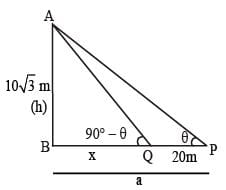In this type of question, just put
h2 = x(x + 20)
⇒ (10√3)2 = x (x + 20)
300 = x (x + 20)
10 (30) = x (x + 20)
x + 20 = 30

SSC CGL Previous Year Questions: Trigonometry - 5 - Question 36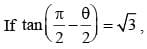value of cos θ is:    (SSC CHSL 2012)

Detailed Solution for SSC CGL Previous Year Questions: Trigonometry - 5 - Question 36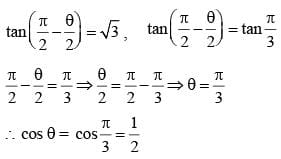SSC CGL Previous Year Questions: Trigonometry - 5 - Question 37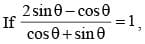then value of cot θ is:   (SSC CHSL 2012)

Detailed Solution for SSC CGL Previous Year Questions: Trigonometry - 5 - Question 37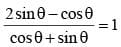Dividing numerator and denominator by cos θ.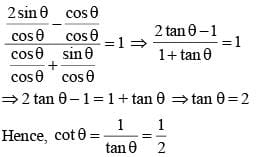SSC CGL Previous Year Questions: Trigonometry - 5 - Question 38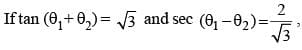then the value of sin 2θ1 + tan 3θ2 is equal to (Assume that 0 < θ1 - θ2 < θ1 + θ2 < 90°)    (SSC Sub. Ins. 2012)

Detailed Solution for SSC CGL Previous Year Questions: Trigonometry - 5 - Question 38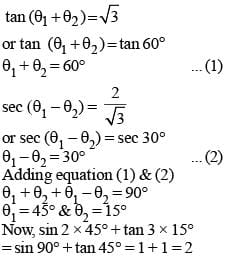SSC CGL Previous Year Questions: Trigonometry - 5 - Question 39

The value of (sec θ – cos θ) (cosec θ – sin θ) (tan θ + cot θ) is   (SSC Sub. Ins. 2012)

Detailed Solution for SSC CGL Previous Year Questions: Trigonometry - 5 - Question 39

(sec θ – cos θ) (cosec θ – sin θ) (tan θ + cot θ)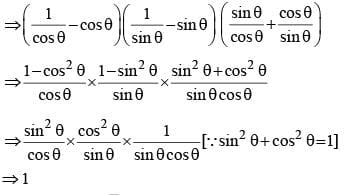SSC CGL Previous Year Questions: Trigonometry - 5 - Question 40

tan 7° tan 23° tan 60° tan 67° tan 83° is equal to   (SSC Sub. Ins. 2012)

Detailed Solution for SSC CGL Previous Year Questions: Trigonometry - 5 - Question 40

tan 7° tan 23° tan 60° tan 67° tan 83°
⇒ tan (90° – 83°) tan (90° – 67°) tan 60° tan 67° tan 83°
⇒ cot 83° cot 67° tan 60° tan 67° tan 83°
[∵ tan (90° – θ) = cotθ]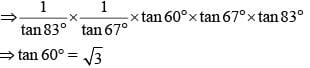SSC CGL Previous Year Questions: Trigonometry - 5 - Question 41

A ladder is resting against a wall at height of 10m. If the ladder is inclined with the ground at an angle of 30°, then the distance of the foot of the ladder from the wall is    (SSC Sub. Ins. 2012)

Detailed Solution for SSC CGL Previous Year Questions: Trigonometry - 5 - Question 41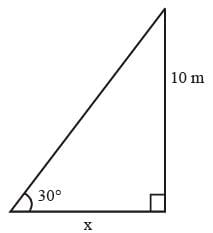Let ‘x’ be the distance of foot of ladder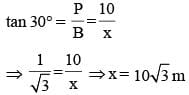## SSC CGL (Tier - 1) - Previous Year Papers (Topic Wise)

250 tests
Information about SSC CGL Previous Year Questions: Trigonometry - 5 Page
In this test you can find the Exam questions for SSC CGL Previous Year Questions: Trigonometry - 5 solved & explained in the simplest way possible. Besides giving Questions and answers for SSC CGL Previous Year Questions: Trigonometry - 5, EduRev gives you an ample number of Online tests for practice

250 tests